Module 25 - Polar Functions

Introduction | Lesson 1 | Lesson 2 | Lesson 3 | Self-Test

Lesson 25.3: Area Bounded by Polar Graphs

Lesson 25.1 introduced polar coordinates and Lesson 25.2 investigated the graphs of polar equations. This lesson explores finding the area bounded by polar graphs.

Finding the Area Bounded by Polar Graphs

The area of the region between the origin and the curve r = f(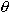) for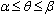is given by the definite integral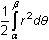The definite integral can be used to find the area of the region enclosed by the cardioid
r = 2(1 + cos).

The entire graph of this function is plotted for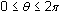, so the area is given by the definite integral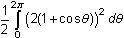Evaluate this integral on your TI-89.

• Enter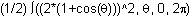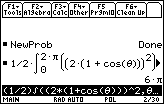The area enclosed by the cardioid is 6square units.

25.3.1 Find the area enclosed by the curve r = 2 on the interval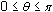. Click here for the answer.

Finding the Area Between Two Polar Curves

The area bounded by two polar curves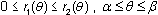is given by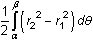The definite integral can be used to find the area that lies inside the circle r = 1 and outside the cardioid r = 1 – cos.

First visualize the area by graphing both curves.

• Set r1 = 1
• Set r2 = 1 – cos()
• Use a [0,2] x [-4,4] x [-2,2] viewing window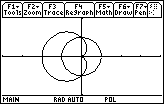The area inside the circle and outside the cardioid lies in the first and fourth quadrants.

To find the area between the curves you need to know the points of intersection of the curves.

•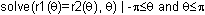• The symbol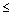is entered by pressing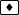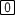.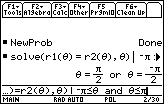It's not always possible to find all of the intersections of two polar curves by simply solving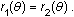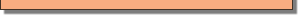The definite integral that gives the area is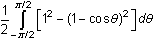• Enter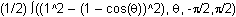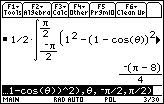The area is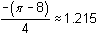square units.

25.3.2 Find the area of the region inside the circle r = 3 sinand outside the cardoid r = 1 + sin. Click here for the answer.

< Back | Next >